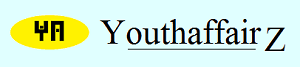November 2012

# Solution

Well, let us consider the number of crows to be "x". The square root of half of them took flight when I approached them. So, the number of crows that took flight is square-root (x/2).

Two crows, at present, have hopped to the side. The rest of the birds, comprising eight-ninths of the flock, are feasting.

Thus, the two birds which hopped to the side and square-root (x/2) number of birds constitute one-ninth of the flock.

Therefore, 2+square-root(x/2) = 1/9*x, that is, 2+square-root(x/2)=x/9.

Therefore, square-root(x/2) = (x/9)-2.

Squaring both sides we get, x/2 = x2/81 - 4x/9 + 4.

Simplifying we get x/2 = (x2 - 36x + 324)/ 81.

That is, 81x = 2x2 - 72x + 648.

That is, 2x2 - 153x + 648 = 0.

Now this is a quadratic equation of the form ax2 + bx + c = 0 whose solutions are: (-b +/- square-root (b2 - 4ac))/2a. So, solving we get x is either 72 or 4.5. But whoever heard of 4.5 birds. The number of crows was, therefore, 72.

• Union Public Service Commission - www.upsc.gov.in
• IIT-Kharagpur - www.iitkgp.ac.in
• Indian Statistical Institute - www.isical.ac.in
• Indian Institute of Technology Madras - www.iitm.ac.in
• Indian Institute of Management, Ahmedabad - www.iimahd.ernet.in
• Indian Institute of Mass Commission - www.iimc.nic.in
• IIT Bombay - www.iitb.ac.in